Worksheets and No Prep Teaching Resources
Critical Thinking Puzzles
Make Puzzles

# Runaway Math Puzzles Puzzle Workbooks for Kids - Updated Each Month!

This page contains Runaway Math Puzzles, which are leveled for second through sixth grade and can be customized with addition, subtraction, multiplication, and division, or any combination thereof. Teachers can also incorporate fractions into the math puzzles. In addition to deciding which math skills to include in the Runaway Math Puzzles, math teachers can also determine how many equations to include in the puzzle. The puzzle can be missing three equations (considered normal difficulty), fewer than three, or more. It can even be missing all the equations (which some consider impossible)! You have full control over all the customization options. These puzzles most closely resemble crossword puzzles, but are completed entirely with equations.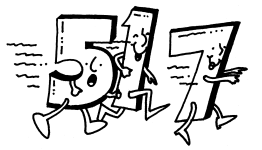Runaway Math Puzzles
Fifth and sixth grade runaway math puzzles
Runaway math puzzles with fractions

Create a custom Runaway Math Puzzle
Create a custom runaway math puzzle
Create a custom runaway math puzzle with missing equations
Create a custom runaway math puzzle where everything is missing

Runaway Math: Three quick pre-made samples for non-subscribers
Pre-made Sample: Normal size and difficulty (all operations)
Pre-made Sample: Missing 3 equations, normal difficulty (all operations)
Pre-made Sample: Super challenging runaway math (everything is missing; all numbers missing; "some" people call this impossible)

Runaway Math: Custom Puzzles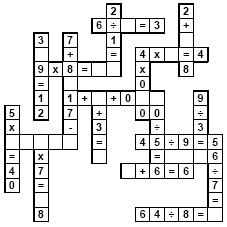Operations
Multiplication and Division
Subtraction only
Multiplication only
Division only

FractionsNo fractions Include fractions (makes puzzle harder)

Difficulty
Easy
Normal
Challenging

Size of Puzzle
Small
Normal
Large
Very Big

Number of Keys (make more keys to have different versions to give out!)
 Select the number of different printables: 1 key 2 keys 3 keys 4 keys 5 keys 6 keys 7 keys 8 keys 9 keys 10 keys

Runaway Math: Missing Equations (you specify how many equations are missing)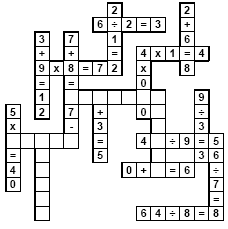Number of Missing Equations
 Select the number of missing equations: 1 missing equation 2 missing equations 3 missing equations 4 missing equations 5 missing equations 6 missing equations 7 missing equations 8 missing equations 9 missing equations 10 missing equations

Operations
Multiplication and Division
Subtraction only
Multiplication only
Division only

FractionsNo fractions Include fractions (makes puzzle harder)

Size of Puzzle
Small
Normal
Large
Very Big

Number of Keys (make more keys to have different versions to give out!)
 Select the number of different printables: 1 key 2 keys 3 keys 4 keys 5 keys 6 keys 7 keys 8 keys 9 keys 10 keys

Super Challenging Runaway Math (everything is missing)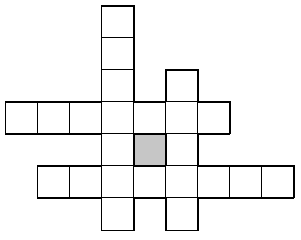Operations
Subtraction only
Multiplication only
Division only
Multiplication and Division

Don't Hide Operations and Equal Signs?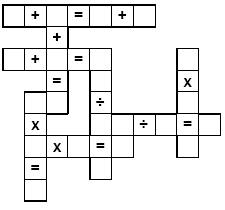Remove all the operations and equal signs also (so everything is blank)
Do not remove the equal signs and operations

FractionsNo fractions Include fractions (makes puzzle harder)

Number of Keys (make more keys to have different versions to give out!)
 Select the number of different printables: 1 key 2 keys 3 keys 4 keys 5 keys 6 keys 7 keys 8 keys 9 keys 10 keys

Teacher Recommendations:  Worksheet and Lesson Plan Activity Ideas

Math

Money

Poetry

Word Search

Puzzles

Science

Main Idea

Weather

Geometry

Probability

Plants

Solar System

Maps

More Activities, Lesson Plans, and Worksheets

Have a suggestion or would like to leave feedback?